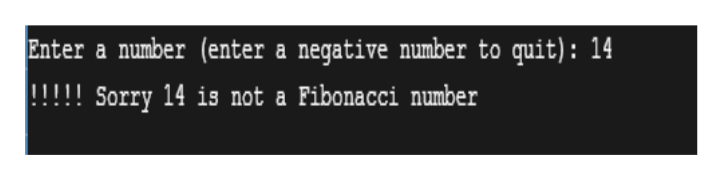# C++  Write a recursive program that finds out if n is a Fibonacci number or not. The Fibonacci numbers are0 1  1          2          3          5          8          13        21        … Your program should have the following interface: Enter a number (enter a negative number to quit): 14!!!!! Sorry14 is not a Fibonacci number Enter a number (enter a negative number to quit): 13Yes, you got it, 13 is a Fibonacci number Enter a number (enter a negative number to quit): 22!!!!! Sorry 22 is not a Fibonacci number Enter a number (enter a negative number to quit): 23!!!!! Sorry 23 is not a Fibonacci number Enter a number (enter a negative number to quit): 21Yes, you got it, 21 is a Fibonacci number Enter a number (enter a negative number to quit):  -1 (*Thanks – Have a good Day*)

Question

C++

Write a recursive program that finds out if n is a Fibonacci number or not. The Fibonacci numbers are

0 1  1          2          3          5          8          13        21        …

Your program should have the following interface:

Enter a number (enter a negative number to quit): 14

!!!!! Sorry14 is not a Fibonacci number

Enter a number (enter a negative number to quit): 13

Yes, you got it, 13 is a Fibonacci number

Enter a number (enter a negative number to quit): 22

!!!!! Sorry 22 is not a Fibonacci number

Enter a number (enter a negative number to quit): 23

!!!!! Sorry 23 is not a Fibonacci number

Enter a number (enter a negative number to quit): 21

Yes, you got it, 21 is a Fibonacci number

Enter a number (enter a negative number to quit):  -1

(*Thanks – Have a good Day*)

check_circleExpert Solution
Step 1

The C++ program will follow the given rubrics:

• Displaying the message to user to enter a number.
• Taking input of number from user.
• If the entered number is negative then terminating the program by using, exit (0) statement.
• If the entered number is valid Fibonacci number, then displaying the number with proper given message.
• If the entered number is equal to 0 or 1, then display that it is a Fibonacci number.
• Else computing the number by putting it in the while loop,

And moving the loop till the value of num2(which is equal to 1 initially) become less than or equal to the user entered value, completing the processing of loop and in last checking that the value of num2 is equal to user entered number or not if yes, then it is a Fibonacci number. Otherwise, the user entered number is not a Fibonacci number.

• If the entered number is not a Fibonacci number, then displaying the number with appropriate given message.
Step 2

Program code:

#include <iostream>

using namespace std;

//main method

int main()

{

//variable declaration

int num, num1 = 0, num2 = 1, temp;

// getting input from user

cout<<"Enter a number (enter a negative number to quit): ";

cin>>num;

//if the number entered by user is negative then exit the program

if (num<0)

{

//exit statement

exit(0);

}

// 0 and 1 are fibonacci numbers

if (num1==num || num2==num)

{

cout<<"%d is a fibonacci number\n"<< num;

return 0;

}

// checking whether a given number is Fibonacci or not

while (num2 <= num)

{

//assigning the value of second number to temp variable

temp = num2;

//assigning the sum value of num1 and num2 to num2

num2 = num1 + num2;

//assigning temp varible value to num1

num1 = temp;

//if num2 is equal to the number entered by user

if (num2 == num)

{

break;

}

}

// printing the results

if (num1==num||num2==num)

{

//displaying message to user that entered number is a fibonacci number

cout<<"Yes, you got it, " <<num<< " is a Fibonacci number";

}

else

{

//displaying message to user that the entered number is not a fibonacci number

cout<<"!!!!! Sorry "<<num <<" is not a Fibonacci number";

}

return 0;

}

Step 3

Output of the above given program code:

1) If user ente...help_outlineImage TranscriptioncloseEnter a number (enter a negative number to quit): 14 Sorry 14 is not a Fibonacci number fullscreen

### Want to see the full answer?

See Solution

#### Want to see this answer and more?

Solutions are written by subject experts who are available 24/7. Questions are typically answered within 1 hour*

See Solution
*Response times may vary by subject and question
Tagged in

### Programing Language